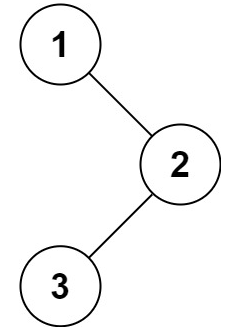# 144.二叉树的前序遍历

## 题目描述``````输入：root = [1,null,2,3]

``````

``````输入：root = []

``````

``````输入：root = 

``````

## 代码

``````# Definition for a binary tree node.
# class TreeNode:
#     def __init__(self, val=0, left=None, right=None):
#         self.val = val
#         self.left = left
#         self.right = right
class Solution:
def preorderTraversal(self, root: Optional[TreeNode]) -> List[int]:

if not root:
return []
res = []
res.append(root.val)
res.extend(self.preorderTraversal(root.left))
res.extend(self.preorderTraversal(root.right))
return res
``````

`extend()` - 用于在列表末尾一次性追加另一个序列中的多个值（用新列表扩展原来的列表）

`list.extend(seq)`： seq – 元素列表

THE END